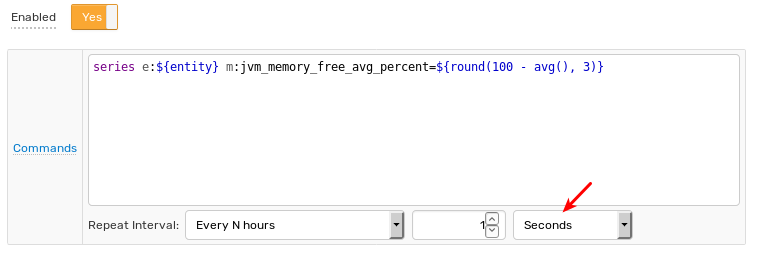# # Derived Commands

## # Overview

Derived command actions store new calculated metrics in the database by creating and processing custom commands defined by Network API syntax.

## # Command Template

To configure a command action, specify a template which contains a command name, command fields and command values.

### # Fields

Command templates can include plaintext and placeholders.

``````series e:\${entity} m:jvm_memory_free_avg_percent=\${round(100 - avg(), 3)}
``````

Calculated metrics can reference other metrics using `db_last`, `db_statistic`, and `value` functions.

``````series e:\${entity} m:jvm_memory_used_bytes=\${value * db_last('jvm_memory_total_bytes') / 100.0}
``````
``````series e:\${entity} m:\${metric}_percent=\${value / value('total') * 100.0} ms:\${command_time.millis}
``````

### # Tags

A special placeholder `\${commandTags}` is provided to print out all command tags in the Network API syntax. Use it to append all tags to the command without knowing tag names in advance.

``````series e:\${entity} m:disk_free=\${100 - value} \${commandTags} ms:\${command_time.millis}
``````

The above expression transforms the input command into a derived command as follows:

Input:

``````series e:test m:disk_used=25 t:mount_point=/ t:file_system=sda ms:1532320900000
``````

Derived:

``````series e:test m:disk_free=75 t:mount_point=/ t:file_system=sda ms:1532320900000
``````

In addition to including specific command tags by name, use the `\${commandTags}` placeholder to copy all tags in the received command.

Command Example
Input `series e:server-01 m:du=25 t:mp=/ t:file_system=sda`
Template `series e:\${entity} m:df=\${100 - value} \${commandTags}`
Derived `series e:server-01 m:df=75 t:mp=/ t:file_system=sda`

### # Time

#### # Current Server Time

To store derived commands with current server time, omit date fields (`ms`, `s`, `d`) from the derived command.

``````series e:\${entity} m:disk_free=\${100 - value} \${commandTags}
``````

Alternatively, use the `now` placeholder to access current server time.

``````series e:\${entity} m:disk_free=\${100 - value} \${commandTags} ms:\${now.millis}
``````

To store commands with second precision, round the current time using `floor` function and `s:` seconds parameter:

``````series e:\${entity} m:disk_free=\${100 - value} \${commandTags} s:\${floor(now.millis/1000)}
``````

#### # Received Time

To store a derived command with the same time as an incoming command, set `ms:` millisecond parameter to `\${command_time.millis}`. This placeholder represents the timestamp of the command that triggered the window status event.

``````series e:\${entity} m:disk_free=\${100 - value} \${commandTags} ms:\${command_time.millis}
``````

command_time Value

If Check On Exit setting is enabled and the condition check is caused by a removed command, the `command_time` field contains the timestamp of the exiting command (oldest command), rounded to seconds.

To round the input time to seconds, use `s:` seconds parameter and `floor` function:

``````series e:\${entity} m:disk_free=\${100 - value} \${commandTags} s:\${floor(command_time.millis/1000)}
``````

## # Frequency

Store derived commands each time a command is received or removed from the window by setting the Repeat parameter to All.

Decrease frequency by adjusting the repeat interval.Produced commands are queued in memory and persisted to the database once per second.

## # Multiple Commands

Specify multiple commands, including those of different types, at the same time. Each command must be specified on a separate line.

``````series e:\${entity} m:jvm_memory_free_avg_percent=\${round(100 - avg(), 3)}
series e:\${entity} m:jvm_memory_free_min_percent=\${round(100 - max(), 3)}
``````

To create multiple metrics within the same command, use a `for` loop to iterate over a collection or array.

``````series e:\${entity} @{s = ""; for (stat : stats) {s = s + " m:" + stat.split(":") + "=" + stat.split(":");} return s;}
``````

If the `stats` collection is `['a:10', 'b:20', 'c:30']`, the produced command is inserted as shown below:

``````series e:entity1 m:a=10 m:b=20 m:c=30
``````

## # Condition

If the purpose of a rule is to create derived series, without any alerting, set the `Condition` field to a static `true` value to minimize processing overhead.## # Examples

### # Moving Average, Last `N` Count

• Window Size: `count = 10`

• Condition: `true`

• Derived Command:

``````series e:\${entity} m:\${metric}_movavg=\${avg()} \${commandTags}
``````

### # Moving Average, Last `N` Time

• Window Size: `time = 10 minute`

• Condition: `true`

• Derived Command:

``````series e:\${entity} m:\${metric}_movavg=\${avg()} \${commandTags}
``````

### # Roll Up (All Matching Entities)

• Window Type: `time: 1 minute`

• Group by Entity: Not Enabled

• Condition: `true`

• Derived Command:

``````series e:total m:\${metric}_sum=\${sum()}
``````

### # Reverse / Inverse Metric

• Window Type: `count = 1`

• Condition: `true`

• Derived Command:

``````series e:\${entity} m:\${metric}_rev=\${100-value} \${commandTags}
``````
``````series e:\${entity} m:\${metric}_inv=\${value = 0 ? 0 : 1/value} \$  {commandTags}
``````

### # Ratio / Percentage

• Window Type: `count = 1`

• Condition: `true`

• Derived Command:

``````series e:\${entity} m:\${metric}_percent=\${100 * value/value('total')} \$  {commandTags}
``````

### # Message to Series

• Window Type: `count = 1`

• Condition: `true`

• Derived Command:

``````series e:\${entity} m:job_execution_time=\$  {tags.job_execution_time.replaceAll("[a-zA-Z]", "").trim()}
``````